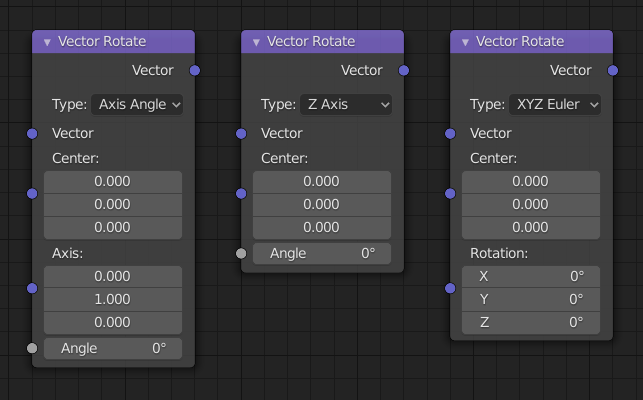# Vector Rotate Node¶Vector Rotate Node.

The Vector Rotate Node provides the ability to rotate a vector around a pivot point (Center).

Vector

Vector to be rotated.

Center (Centro)

Point to rotate around.

## Properties¶

Type (Tipo)

The type of angle input.

X, Y, Z Axis

Rotates the vector around the defined axis and the amount of rotation is defined by the Angle input.

Axis Angle

Rotates the vector around any arbitrary axis defined by the Axis input vector. The amount of rotation is defined by the Angle input.

Euler

Rotates the vector about the Center with the Rotation input vector to control the amount of rotation on each axis.

Invert (Invertir)

Inverts the rotation angle.

## Salidas¶

Vector

The rotated vector.

## Ejemplos¶Vector Rotate node example.